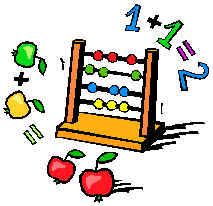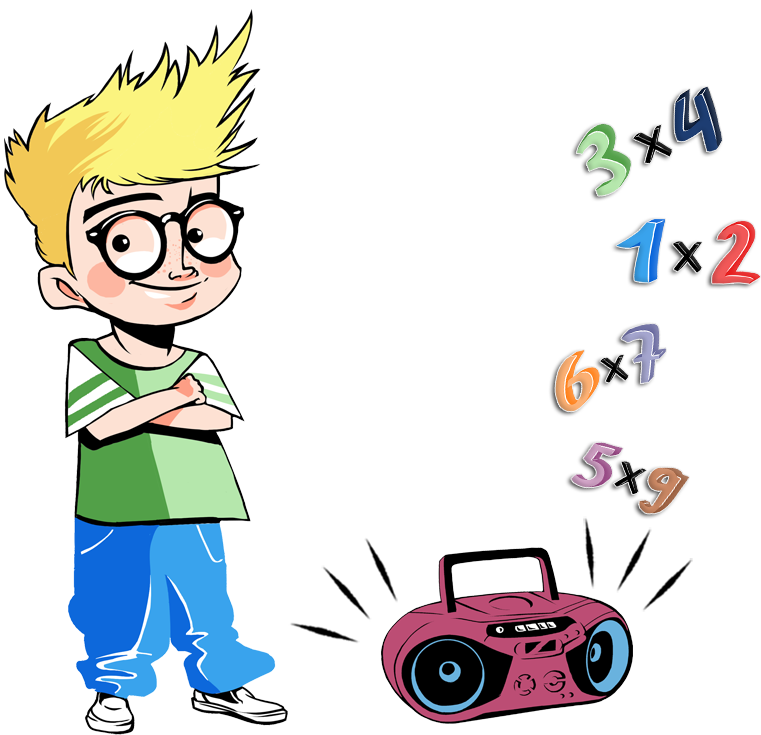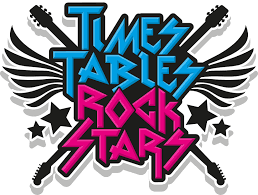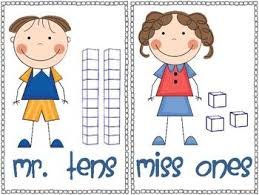# Year 2Calculation videos

Please click on the calculation areas at the side to see step by step videos of the methods we use for Addition, Subtraction, Multiplication and Division  in our Maths lessons. These videos are useful for both parents and children.

We are adding new videos all the time, so please keep checking back.Useful websites

The following links will help you to support your child's learning at home and link to their maths targets.TargetI can count in tens from any number, forward and back.

Finding 10 more or less activity

Target - I can compare and order numbers to 100 and use <, > and = signs.

Learn all about numbers to 100

Learn how to order numbers to 100 part 1

Ordering numbers activity

Target - I can count in 2s, 3s and 5s.Target - I can understand the words sum and difference.

Subtraction recap

Subtraction vocabulary

Finding the difference activity

Target - I can add and subtract a 2 digit and 2 digit number in my head.

Learn how to add 2 digit numbers together

Addition and subtraction activity

Target - I can add three 1 digit numbers in my head.Target - I can say the 2, 3, 5 and 10 times table.

I can say the related division facts.

Introducing multiplication

Target - I can solve multiplication problems in my head.

Learn how to solve multiplication problemsTT Rock Stars is great way to practise your times tables and enter competitions.

TT Rock StarsTarget - I can say 10 more or 10 less than a number.

Learn how to find 10 more or less

Finding 10 more or less activity

Target - I can recognise the place value of each digit in two-digit numbers (tens and ones).

Learn how to identify tens and ones in a two-digit number

Partitioning two-digit numbers (Part 1)

Partitioning two-digit numbers (Part 2)

Representing two-digit numbers (Part 1)

Representing two-digit numbers (Part 2)

Target - I can read and write numbers to 100 in numerals and words.

Learn how to write numbers as words

Matching numbers to words activity

Spelling numbers activity

Daily maths challengeIf you would like to have a go at a daily maths challenge click on the link below.

Daily 10 challenge

Select level two and choose your challenge from one the following options: